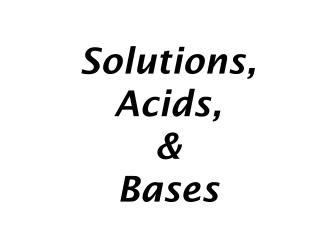DownloadDownload PresentationSolutions, Acids, & Bases

# Solutions, Acids, & Bases

Télécharger la présentation## Solutions, Acids, & Bases

- - - - - - - - - - - - - - - - - - - - - - - - - - - E N D - - - - - - - - - - - - - - - - - - - - - - - - - - -
##### Presentation Transcript

1. Solutions,Acids,&Bases

2. Solutions

3. Solutions Solutions • type of homogeneous mixture (no visibly different particles) • solute is dissolved in a solvent (Not always solid dissolved in a liquid)

4. Solutions Solutions • solubility – how much solute is able to be dissolved in a given solvent • factors • temperature • pressure • surface area • stirring

5. Solutions Solutions • solubility • degrees • unsaturated – more solute may theoretically be dissolved • saturated – maximum theoretical amount of solute is dissolved • supersaturated – more solute dissolved than predicted at given temp.

6. Solutions Solutions • concentration – measure of amount of solute dissolved per quantity of solvent • dilute solution – low amount of solute • concentrated solution – high amount of solute • molarity (M) – moles of solute dissolved per liter of solution • also called molar concentration

7. Solutions Molarity sample problems • A solution has a volume of 2.0 L and contains 36.0 g glucose. If the molar mass of glucose is 180 g/mol, find the molarity.

8. Solutions Molarity sample problems • A saline solution contains 0.90 g NaCl per 100 mL of solution. What is the molarity?

9. Solutions Molarity sample problems • How many moles of solute are present in 1.5 L of 0.24 M Na2SO4?

10. Solutions Molarity sample problems • How many moles of ammonium nitrate are in 335 mL of 0.425 M NH4NO3?

11. Solutions Solutions • concentration • diluting – lowering amount of solute in solution by adding more solvent (usually water) • M1× V1 = M2 × V2

12. Solutions Diluting sample problems • How many milliliters of a stock solution of 4.00 M KI would you need to prepare 250.0 mL of 0. 760 M KI?

13. Solutions Diluting sample problems • How many milliliters of a stock solution of 2.00 M MgSO4 would you need to prepare 100.0 mL of 0.400 M MgSO4?

14. Acids&Bases

15. Acids & Bases Acids • substances that donate hydrogen ions to form hydronium ions (H3O1+) when dissolved in water • strong acids fully ionize in water • weak acids do not fully ionize in water

16. Acids & Bases Bases • substances that either contain hydroxide ions or react with water to form hydroxide ions • strong bases fully ionize in water • weak bases do not fully ionize in water

17. Acids & Bases Acids & Bases • degrees of acidity or basicity • measured by pH • concentration of hydronium ions in solution • pH < 7.0 → acidic • pH = 7.0 → neutral • pH > 7.0 → basic

18. Acids & Bases Acids & Bases • at home… • cleaning products are often basic • food products are often acidic

19. Acids & Bases Acids & Bases • mixing acids & bases may cause neutralization, forming water and a salt • Ex: HCl(aq) + NaOH(aq)NaCl(aq) + H2O